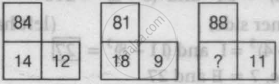# Examine the Following Three Figures in Which the Numbers Follow a Specific Pattern. the Missing Number (?) in the Third Figure Above is - Logical Reasoning

MCQ

Examine the following three figures in which the numbers follow a specific pattern.The missing number (?) in the third figure above is

• 7

• 16

• 21

• 28

#### Solution

16
Explanation:

In the first two figures, the number at the top is divided by the number at the right and is then doubled. As in the first figure, 84/12=7 and

2 x 7 = 14. In the second figure, 81/9=9 and

2 x 9 = 18. In the third figure,88/11=8. And the missing number will be 2 x 8 = 16

Concept: Inserting the Missing Character (Entrance Exam)
Is there an error in this question or solution?